# Maharashtra Board 11th Maths Solutions Chapter 4 Determinants and Matrices Ex 4.4

Balbharti Maharashtra State Board Class 11 Maths Solutions Pdf Chapter 4 Determinants and Matrices Ex 4.4 Questions and Answers.

## Maharashtra State Board 11th Maths Solutions Chapter 4 Determinants and Matrices Ex 4.4

Question 1.
Construct a matrix A = [aij]3 x 2 whose elements ay are given by
i. aij = $$\frac{(\mathbf{i}-\mathbf{j})^{2}}{5-\mathbf{i}}$$
ii. aij = i – 3j
iii. aij $$\frac{(i+j)^{3}}{5}$$
Solution: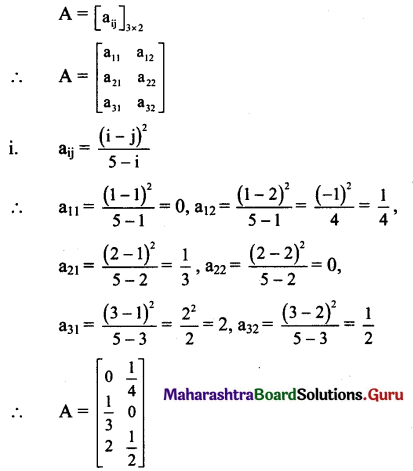[Note: Answer given in the textbook is A = $$\left[\begin{array}{ll} 0 & \frac{1}{4} \\ \frac{1}{2} & 0 \\ 2 & \frac{1}{2} \end{array}\right]$$
However, as per our calculation it is $$\left[\begin{array}{ll} 0 & \frac{1}{4} \\ \frac{1}{3} & 0 \\ 2 & \frac{1}{2} \end{array}\right]$$ ].ii. aij = i – 3j
∴ a11 = 1 – 3(1) = 1 – 3 = -2,
a12= 1 – 3(2) = 1 – 6 = -5,
a21 = 2 – 3(1) = 2 – 3 =-1,
a22 = 2 – 3(2) = 2 – 6 = – 4
a31 = 3 – 3(1) = 3-3 = 0,
a32 = 3 – 3(2) = 3 – 6 = -3
∴ A = $$\left[\begin{array}{cc} -2 & -5 \\ -1 & -4 \\ 0 & -3 \end{array}\right]$$

iii. aij = $$\frac{(i+j)^{3}}{5}$$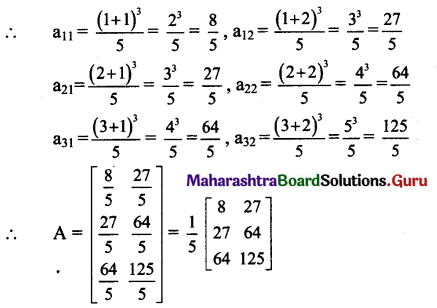Question 2.
Classify the following matrices as a row, a column, a square, a diagonal, a scalar, a unit, an upper triangular, a lower triangular, a symmetric or a skew- symmetric matrix.
i. $$\left[\begin{array}{ccc} 3 & -2 & 4 \\ 0 & 0 & -5 \\ 0 & 0 & 0 \end{array}\right]$$
Solution:
Let A = $$\left[\begin{array}{ccc} 3 & -2 & 4 \\ 0 & 0 & -5 \\ 0 & 0 & 0 \end{array}\right]$$
As every element below the diagonal is zero in matrix A.
∴ A is an upper triangular matrix.

ii. $$\left[\begin{array}{ccc} 0 & 4 & 7 \\ -4 & 0 & -3 \\ -7 & 3 & 0 \end{array}\right]$$
Solution:
Let A = $$\left[\begin{array}{ccc} 0 & 4 & 7 \\ -4 & 0 & -3 \\ -7 & 3 & 0 \end{array}\right]$$
∴ AT = $$\left[\begin{array}{ccc} 0 & -4 & -7 \\ 4 & 0 & 3 \\ 7 & -3 & 0 \end{array}\right]$$
∴ AT = $$-\left[\begin{array}{ccc} 0 & 4 & 7 \\ -4 & 0 & -3 \\ -7 & 3 & 0 \end{array}\right]$$
∴ AT = -A, i.e., A = -AT
∴ A is a skew-symmetric matrix.iii. $$\left[\begin{array}{c} 5 \\ 4 \\ -3 \end{array}\right]$$
Solution:
Let A = $$\left[\begin{array}{c} 5 \\ 4 \\ -3 \end{array}\right]$$
∴ As matrix A has only one column.
∴ A is a column matrix.

iv. $$\left[\begin{array}{lll} 9 & \sqrt{2} & -3 \end{array}\right]$$
Solution:
Let A = $$\left[\begin{array}{lll} 9 & \sqrt{2} & -3 \end{array}\right]$$
As matrix A has only one row.
∴ A is a row matrix.

v. $$\left[\begin{array}{ll} 6 & 0 \\ 0 & 6 \end{array}\right]$$
Solution:
Let A = $$\left[\begin{array}{ll} 6 & 0 \\ 0 & 6 \end{array}\right]$$
As matrix A has all its non-diagonal elements zero and diagonal elements same.
∴ A is a scalar matrix.

vi. $$\left[\begin{array}{ccc} 2 & 0 & 0 \\ 3 & -1 & 0 \\ -7 & 3 & 1 \end{array}\right]$$
Solution:
Let A = $$\left[\begin{array}{ccc} 2 & 0 & 0 \\ 3 & -1 & 0 \\ -7 & 3 & 1 \end{array}\right]$$
As every element above the diagonal is zero in matrix A.
∴ A is a lower triangular matrix.

vii. $$\left[\begin{array}{ccc} 3 & 0 & 0 \\ 0 & 5 & 0 \\ 0 & 0 & \frac{1}{3} \end{array}\right]$$
Solution:
Let A = $$\left[\begin{array}{ccc} 3 & 0 & 0 \\ 0 & 5 & 0 \\ 0 & 0 & \frac{1}{3} \end{array}\right]$$
As matrix A has all its non-diagonal elements zero.
∴ A is a diagonal matrix.viii. $$\left[\begin{array}{ccc} 10 & -15 & 27 \\ -15 & 0 & \sqrt{34} \\ 27 & \sqrt{34} & \frac{5}{3} \end{array}\right]$$
Solution: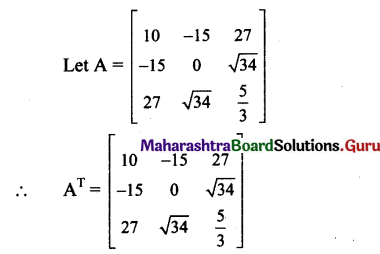∴ AT = A, i/e., A = AT
∴ A is a symmetric matrix.

ix. $$\left[\begin{array}{lll} 1 & 0 & 0 \\ 0 & 1 & 0 \\ 0 & 0 & 1 \end{array}\right]$$
Solution:
A = $$\left[\begin{array}{lll} 1 & 0 & 0 \\ 0 & 1 & 0 \\ 0 & 0 & 1 \end{array}\right]$$
In matrix A, all the non-diagonal elements are zero and diagonal elements are one.
∴ A is a unit (identity) matrix.

x. $$\left[\begin{array}{lll} 0 & 0 & 1 \\ 0 & 1 & 0 \\ 1 & 0 & 0 \end{array}\right]$$
Solution:
Let A = $$\left[\begin{array}{lll} 0 & 0 & 1 \\ 0 & 1 & 0 \\ 1 & 0 & 0 \end{array}\right]$$
∴ AT = A, i/e., A = AT
∴ A is a symmetric matrix.
∴ A is a symmetric matrix.

Question 3.
Which of the following matrices are singular or non-singular?
i. $$\left[\begin{array}{ccc} \mathbf{a} & \mathbf{b} & \mathbf{c} \\ \mathbf{p} & \mathbf{q} & \mathbf{r} \\ \mathbf{2 a}-\mathbf{p} & \mathbf{2 b}-\mathbf{q} & \mathbf{2 c}-\mathbf{r} \end{array}\right]$$
ii. $$\left[\begin{array}{ccc} 5 & 0 & 5 \\ 1 & 99 & 100 \\ 6 & 99 & 105 \end{array}\right]$$
iii. $$\left[\begin{array}{ccc} 3 & 5 & 7 \\ -2 & 1 & 4 \\ 3 & 2 & 5 \end{array}\right]$$
iv. $$\left[\begin{array}{cc} 7 & 5 \\ -4 & 7 \end{array}\right]$$
Solution: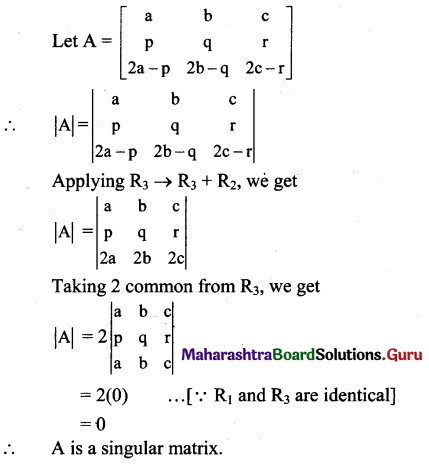ii. Let A = $$\left[\begin{array}{ccc} 5 & 0 & 5 \\ 1 & 99 & 100 \\ 6 & 99 & 105 \end{array}\right]$$
∴ |A| = $$\left|\begin{array}{ccc} 5 & 0 & 5 \\ 1 & 99 & 100 \\ 6 & 99 & 105 \end{array}\right|$$
Applying C2 → C2 + C1
|A| = $$\left|\begin{array}{ccc} 5 & 5 & 5 \\ 1 & 100 & 100 \\ 6 & 105 & 105 \end{array}\right|$$
= 0 … [∵ C2 and C3 are identical]
∴ A is a singular matrix.

iii. Let A = $$\left[\begin{array}{ccc} 3 & 5 & 7 \\ -2 & 1 & 4 \\ 3 & 2 & 5 \end{array}\right]$$
∴ |A| = $$\left|\begin{array}{ccc} 3 & 5 & 7 \\ -2 & 1 & 4 \\ 3 & 2 & 5 \end{array}\right|$$
= 3(5 – 8) – 5(-10 – 12) + 7(-4 – 3)
= -9 + 110 – 49 = 52 ≠ 0
∴ A is a non-singular matrix.

iv. Let A = $$\left[\begin{array}{cc} 7 & 5 \\ -4 & 7 \end{array}\right]$$
∴ |A| = $$\left[\begin{array}{cc} 7 & 5 \\ -4 & 7 \end{array}\right]$$ = 49 + 20 = 69 ≠ 0

Question 4.
Find k, if the following matrices are singular.
i. $$\left[\begin{array}{cc} 7 & 3 \\ -2 & k \end{array}\right]$$
ii. $$\left[\begin{array}{ccc} 4 & 3 & 1 \\ 7 & k & 1 \\ 10 & 9 & 1 \end{array}\right]$$
iii. $$\left[\begin{array}{ccc} k-1 & 2 & 3 \\ 3 & 1 & 2 \\ 1 & -2 & 4 \end{array}\right]$$
Solution:
Let A = $$\left[\begin{array}{cc} 7 & 3 \\ -2 & k \end{array}\right]$$
Since A is a singular matrix,
|A|=0
∴ $$\left|\begin{array}{cc} 7 & 3 \\ -2 & \mathrm{k} \end{array}\right|$$ = o
∴ 7k + 6 = 0
∴ 7k = -6
k = -6/7

ii. Let A = $$\left[\begin{array}{ccc} 4 & 3 & 1 \\ 7 & k & 1 \\ 10 & 9 & 1 \end{array}\right]$$
Since A is a singular matrix,
|A|= 0
∴ $$\left|\begin{array}{ccc} 4 & 3 & 1 \\ 7 & \mathrm{k} & 1 \\ 10 & 9 & 1 \end{array}\right|$$ = 0
∴ 4(k – 9) – 3(7 – 10) + 1(63 – 10k) = 0
∴ 4k – 36 + 9 + 63 – 10k = 0
∴ -6k + 36 = 0
∴ 6k = 36
∴ k = 6

iii. Let A = $$\left[\begin{array}{ccc} \mathbf{k}-1 & 2 & 3 \\ 3 & 1 & 2 \\ 1 & -2 & 4 \end{array}\right]$$
Since A is a singular matrix
|A| = 0
∴ $$\left|\begin{array}{ccc} k-1 & 2 & 3 \\ 3 & 1 & 2 \\ 1 & -2 & 4 \end{array}\right|$$
∴ (k – 1)(4 + 4) – 2(12 – 2) + 3 (-6 – 1) = 0
∴ 8k-8-20-21 =0
∴ 8k = 49
∴ k = 49/8Question 5.
If A = $$\left[\begin{array}{lll} 5 & 1 & -1 \\ 3 & 2 & 0 \end{array}\right]$$, find (AT)T.
Solution: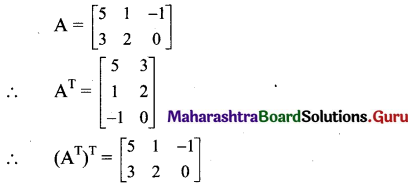Question 6.
If A = , find (AT)T.
Solution: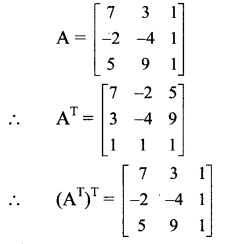Question 7.
Find a, b, c, if $$\left[\begin{array}{ccc} 1 & \frac{3}{5} & a \\ b & -5 & -7 \\ -4 & c & 0 \end{array}\right]$$ is a symmetric matrix.
Solution: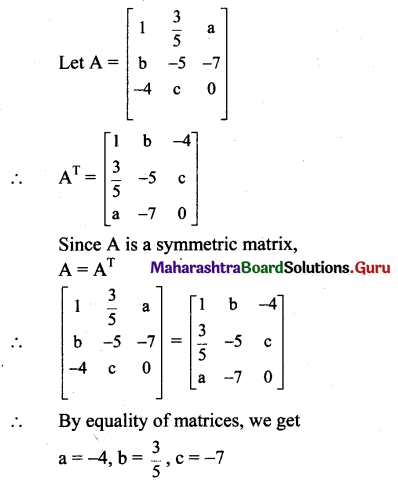Question 8.
Find x, y, z, if  is a symmetric matrix.
Solution: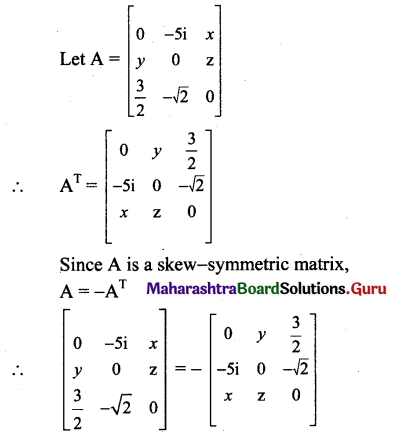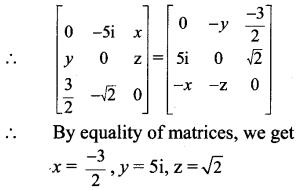Question 9.
For each of the following matrices, using its transpose, state whether it is symmetric, skew-symmetric or neither.
i. $$\left[\begin{array}{ccc} 1 & 2 & -5 \\ 2 & -3 & 4 \\ -5 & 4 & 9 \end{array}\right]$$
ii. $$\left[\begin{array}{ccc} 2 & 5 & 1 \\ -5 & 4 & 6 \\ -1 & -6 & 3 \end{array}\right]$$
iii. $$\left[\begin{array}{ccc} 0 & 1+2 \mathbf{i} & \mathbf{i}-2 \\ -1-2 \mathbf{i} & 0 & -7 \\ 2-\mathbf{i} & 7 & 0 \end{array}\right]$$
Solution:
i. Let A = $$\left[\begin{array}{ccc} 1 & 2 & -5 \\ 2 & -3 & 4 \\ -5 & 4 & 9 \end{array}\right]$$
∴ AT =$$\left[\begin{array}{ccc} 1 & 2 & -5 \\ 2 & -3 & 4 \\ -5 & 4 & 9 \end{array}\right]$$
∴ AT = A, i.e., A = AT
∴ A is a symmetric matrix.

ii.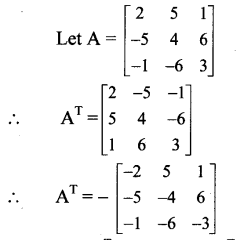∴ A ≠ AT, i.e., A ≠ -AT
∴ A is neither a symmetric nor skew-symmetric matrix.iii.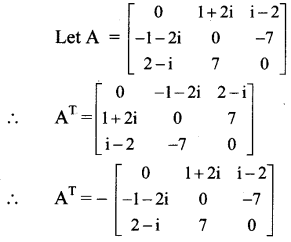∴ AT = -A, i.e., A = -AT
∴ A is a skew-symmetric matrix.

Question 10.
Construct the matrix A = [aij]3 x 3, where aij = i – j. State whether A is symmetric or skew-symmetric.
Solution:
A = [aij]3 x 3
∴ A = $$\left[\begin{array}{lll} a_{11} & a_{12} & a_{13} \\ a_{21} & a_{22} & a_{23} \\ a_{31} & a_{32} & a_{33} \end{array}\right]$$
Given, aij = i – j
a11 = 1-1 = 0, a12 = 1-2 = – 1, a13 = 1 – 3 = – 2,
a21 – 2 – 1 = 1, a22 = 2 – 2 = 0, a23 =2 – 3 = – 1,
a31 = 3 – 1 = 2, a32 = 3 – 2 = 1, a33 = 3 – 3 = 0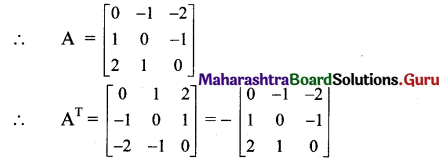∴ AT = -A, i.e., A = -AT
∴ A is a skew-symmetric matrix.